# KSEEB Solutions for Class 5 Maths Chapter 6 Weight and Volume

Students can Download Maths Chapter 6 Weight and Volume Questions and Answers, Summary, Notes Pdf, KSEEB Solutions for Class 5 Maths helps you to revise the complete Karnataka State Board Syllabus and score more marks in your examinations.

## Karnataka State Syllabus Class 5 Maths Chapter 6 Weight and Volume

### KSEEB Class 5 Maths Weight and Volume Ex 6.1

Question 1.
Albert buys 16 kg of jaggery and 15kg of rice from a shop. He puts both the packets into one bag. What is the total weight of the bag?
Albert buys jaggry = 16 K.G
Albert buys Rice = 15 K.G
Albert puts jiggery and rice = 31 K.G in one bag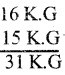Question 2.
62kg of rice was supplied to a school under ‘Aksharadasoha Scheme’. If 15 kg of rice was used, find the quantity of rice remaining.
Akshara dasoha supplied to school – 62 KG
The school used of rice – 15 KG
The remaining quantity of rice – 47 KGQuestion 3.
A bag can hold 20kg of soji. If 140 of soji is to be filled equally, how many such bags are required?
A bag can hold of soji = 20 KG
Soji is equally filled = 140KG
The number of bags = $$\frac{140}{20}$$ = 7

Question 4.
A marchant has 5 packets of sugar, each packet weighting 15kg of sugar. What is the total weight of 5 packets of sugar?
A merchant has 5 packets of sugar, Each packet weight of sugar 15 kg
The total weight of 5 packet of sugar = $$\frac{15 \times 5}{75 \mathrm{kgs}}$$

II. Convert the following:

1. 7 kg = ______ gram.
1 kg = 1000 gram
7 kg = 1000 × 7
= 7000 gram

2. 16 gram = ______ milligram.
1 gram = 1000 mg
16 gram = 1000 × 16
= 16000 milligrams

3. 6000 gram = _______ kg.
1 kg = 1000 gram
6000gram = $$\frac{6000}{1000}$$ = 6kg.

4. 750 milligram = ______ gram.
1 grams = 1000 milligrams
750 milligrams = $$\frac{750}{100}$$ = 7.5grams

5. 12 quintals = ________ kg.
1 quintals = 100 kg
12 quintals = 12 × 100 = 1200 kg6. 1,500 kg = _______ quintals.
1 quintals = 100 kg
1500 kg = $$\frac{1500}{100}$$= 15 quintals

1. 4kg, 250g, 12kg and 355g.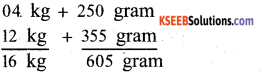2. 23kg, 432g,37 kg and 350g.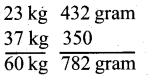3. 12kg, 450g, 15kg and 550g.4. 17kg, 370g, 18kg and 630g.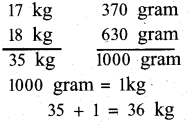5. 6kg 250g, 8kg 430g, 7kg 220g and 430g.IV. Subtract the fallowing:

1. 13kg 250g from 28kg 550g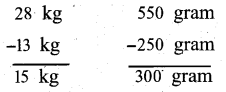2. 35kg 550g from 70kg 675g3. 55kg 335g from 85kg 730g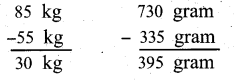4. 28kg 650g from 63 kg 350g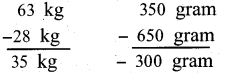5. 34 kg 585g form 75kg 475gV. Solve the following:

Question 1.
Radha buys 2kg 500g of carrot, 2kg 5Q0g of brinjal and 3kg 250g of tomatoes from a vegetable shop. Find the total weight of vegetables bought by her.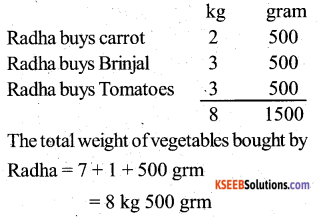Question 2.
A merchant has 68kg 250g of wheat with him. He sells 15kg 250g of wheat to one customer and 13kg 500g to another customer. What is the weight of wheat remaning with him?
A merchant has 68 kg+250 grm of wheat ,(one customer) A merchant has soldi5kg 250gm. (Another customer) A merchant has sold 13kg500gm.The remaining wheat of a merchant 39 kg 500 gram

Question 3.
The yield of paddy per acre is 29 quintals and 50kg. Rachappa has 30 acres of field. What is the total yield of paddy?
The yield of paddy per acre = 29 quintals and 50kgThe total yield of paddy = 8 8,5 00 kgsQuestion 4.
18kg 400g of sugar is to be packed in 4 bags equally. What is the weight of each bag?
1kg = 100 grm
8 kg = 1000 × 18 = 18,000 kgs
18 kg + 400 grm of sugar is to be packed in 4 bags equally = $$\frac{18400}{4}$$ = 4,600 grm
The weight of each bag 4,600 grm

Question 5.
A farmer gets 60 to 400 g of onion from one field and 56kg 800g from another field. If he sells 98kg 200g of onion, find the weight of onions left with him.
From a land, A farmer 60kg+400 grm’of onion From another land, A farmer 56 kg + 800 grm of onionThe weight of onion 19 kg left with him

### KSEEB Class 5 Maths Weight and Volume Ex 6.2

Question 1.
A glass contains 300ml of water and another glass contains 250ml of water. If water from both the glasses are poured into a jug, what is the quantity of water in the jug?
A glass contains of water 300 ml
Another glass contains of water 250ml
The glasses ofwater poured into 300 + 250 = 550 ml

Question 2.
A milkman has 5l700ml of milk. Out of this he sells 11350ml of milk to one person and 21250ml to another person. Find the quantity of milk left with the milkman.
A milk man has 5l700 ml of milk
For one person, A milkman sells 11350 ml
For another person A milkman sells 21250 ml
The total selling of milk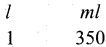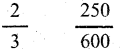The quantity of milk left with milkman =?The quantity of milk left with milkman 2l 100 ml

Question 3.
A bottle contains 200ml of milk. What is the total quantity of milk contained in 5 such bottles?
A bottle contains of milk 200 ml
5 such bottles of milk = 200 × 5 = 1000 ml
The total quantity of milk 1000 ml (or) 1l

Question 4.
100 ml of sugarcane juice is distrubuted among 4 friends equally. What quantity of sugarcane juice does each one get?
There are 4 friends
Sugarcane juice=100 ml
The total quantity of milk= $$\frac{100}{4}$$ = 25 = 25ml

II. Convert the following:

1. 5l = 5000 ml.
1l = 1000 ml
5l = 1000 × 5
= 5000 ml2. 6ml = 600 l.
1 l = 1000 ml
6l = 1000 × 6 = 6000 ml

3. 500 ml. = 1/2l
1 l = 1000 ml.
500ml = $$\frac{500}{1000}$$ = 1/2

4. 8,000 l = 8 kilolitre
1 l = 1000 ml
8000l = $$\frac{8000}{1000}$$ = 8l.

1. 3l 250ml, 750 ml and 6l 500 ml.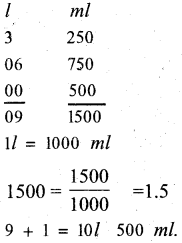2. 15l 450ml, 5l 350ml and 21l 750ml.3. 12l. 550ml, 14l 450ml and 12l 650ml.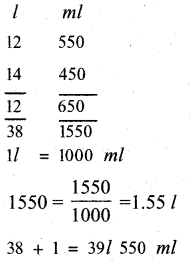4. 8kl 250l, 3kl 350l and 4kl 550l.IV. Subtract:

1. 15l 750 ml from 25l 350 ml2. 24l 550ml from 64l 450ml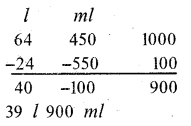3. 45l 800 ml from 75l 750 ml4. 34 kilolitre 750l from 55 kilolitre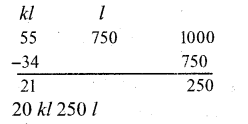V. Solve the following:

Question 1.
Veena buys 3l 250 ml of groundnut oil while returning from her office. Her mother also bought 1l 500 ml of groundnut oil on the same day. What is the total quantity of groundnut oil bought by them?
Veena buys of groundnut oil – 3(l), 250(ml)
Veena’s mother buys of groundnut oil – 1(l), 500(ml)
The total quantity of groundnut oil=4l, 750ml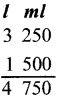Question 2.
A milkman has 25 litres of milk. He sells 16l 750 ml of it. Find the quantity of milk left with him.
A milkman has 25 litres of milk.
He sells of milk 16l750 ml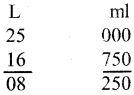The quantity of milk left 8 litres 250ml

Question 3.
A bottle contains 1l 500 ml of sandal oil. It has to be filled into bottles of 250 ml each. How many bottles are required?

A bottle contains of sandal oil 1 liter 500 ml
For each Bottle 250 ml fill
The no of Bottles = ?
ll = 1000 ml
1 l + 500 ml = 1500 ml
The no of bottle = $$\frac{1500}{250}$$ = 6.

Question 4.
An oil can contains 15l of Sunpure oil. Out of this Ram uses 3/ 600 ml in the first month and 5/ 300 ml in the sec ond month. Find the quantity of oil left in the oil can.
An oil can contains 15 l of sunpure
Rani uses in first month 31600 ml
Rani uses in second month 51300 mlThe total oil used by Rani in 2 months 8l 900 ml
The quantity of oil left with Rani l ml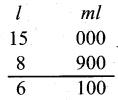Question 5.
A vessel contains l litre 800 ml of tea. It is poured into 12 glasses of thesame size equally. What is the quantity of tea contained in each glass?
12 such glasses = $$\frac{180}{12}$$ = 150ml.